# PSO Based Algorithm for Optimal PMU Placement in KPTC

3880 words (16 pages) Essay

28th Sep 2017 Engineering Reference this

Tags:

Disclaimer: This work has been submitted by a university student. This is not an example of the work produced by our Essay Writing Service. You can view samples of our professional work here.

Any opinions, findings, conclusions or recommendations expressed in this material are those of the authors and do not necessarily reflect the views of UKEssays.com.

A PSO Based Cluster Formation Algorithm for Optimal PMU Placement in KPTCL

First A. Author, Designation, Organization, Second B. Author, and Third C. Author, Jr., Designation, Organization

Abstract Power system state estimation with the exclusive deployment of synchronous phasor measurements demands that the system must be completely observable with PMUs only. Direct measurement of phase angles of current and voltage phasors are now possible by Phasor Measurement Units (PMUs). To have lesser number of PMUs, the placement problem in any network is considered as an optimization problem. This paper presents a Particle Swarm Optimization (PSO) based cluster formation algorithm for optimal PMU placement. The proposed algorithm clusters the buses into many sub groups and the maximum connectivity bus is selected as the header bus. The PMU is placed on the header bus to manage the connected buses for complete system observability. This paper analyses the proposed algorithm for the following three cases:

1. Without PMU loss,
2. With single PMU loss, and
3. Zero Injection Bus.

The simulation results for IEEE bus and the KPTCL bus systems are presented and compared with the existing approaches. The proposed results show that the method is simple to implement and provide the accurate PMU placement.

Index Terms IEEE Bus, Karnataka Power Transmission Corporation Limited (KPTCL), Optimal PMU Placement, Particle Swarm Optimization (PSO), Phasor Measurement Units (PMUs), and Power System State Estimation

## I. Introduction

Power utilities are facing numerous threats of security of operation due to the over stressed power network in the today’s competitive power market scenario. Phasor Measurement Unit (PMU) is an evaluating device which is used to measure the current and voltage. It uses the Global Positioning System (GPS) pulse to facilitate the synchronized measurements of real time phasors of currents and voltage. A power system is said to be recognizable when voltage phasors at all the buses are known. According to Ohm’s Law, if a PMU is placed at the bus, then the neighboring buses also become observable. Obviously, when PMUs are placed at all the buses of the network, and the measurements for all the PMUs are communicated to the control units, then the voltage phasors at all the buses would be known. This approach can change the traditional estimation to state measurement. PMUs are already installed in several utilities for various applications around the world such as state estimation, adaptive protection and system protection schemes. Other application fields include stability monitoring, Wide Area Monitoring and Control (WAMC) and efficient system utilization.

In the traditional power systems, the buses are monitored using the conventional measurements from voltage and current transformers and the data are forwarded to the Energy Management System (EMS) through the Supervisory Control and Data Acquisition (SCADA) system. It collects the real time measurements from the Remote Terminal Units (RTUs) placed in substations. This approaches are not able to monitor all the measurements across a wide area power system because the data are not time-synchronized . PMUs are an essential part of smart grids and hence the rate of PMU installations are increasing. In the emerging technology, the major issue need to be addressed is the placement of PMUs, which is influenced by the anticipated system applications. The major factor limiting the number of PMU installations are their cost and the communication facilities. Hence, the cost and communication constraints of PMUs have been motivated the researchers to identify the minimal PMU installation for the anticipated applications. Placing PMUs on all buses of the power system results a complete observability of the system. Since, a bus is observed if a PMU is placed on it or some of its neighboring buses, it is neither economical nor necessary to carry put such installations. As a consequence, a problem called Optimal PMU Placement (OPP) problem has been occurs.

The aim of this paper is to identify the optimal number of PMUs to make the KPTCL topologically observable. Here, a PSO based Clustering Algorithm is proposed to cluster the buses. The header bus is selected based on the maximum connectivity among the buses. The header bus is placed with the PMU to monitor the other connected buses. The PMU placement strategy confirms the system observability during the normal working conditions and also the single PMU failures. The proposed method is found to be simple, fast and accurate in computation. The proposed method is applied on IEEE-6, IEEE-7, IEEE-9, IEEE-14, IEEE-30 bus systems and KPTCL power maps for 28 bus, 127 bus and 155 bus systems to verify the proposed algorithm performance.

The remaining part of the paper is organized as follows: Section II involves the works related to the existing algorithms for optimal PMU placement problem. Section III involves the description of the proposed PSO based cluster formation algorithm for optimal PMU placement. Section IV involves the performance analysis of the proposed work. The paper is concluded in Section V.

## II. Related Works

With the number of PMUs estimated for installation in the near future, both the utilities and researchers are looking for the optimal solutions to their placement. The solutions for the optimal PMU placement problem can be classified into two types: mathematical and heuristic algorithms. Some of the existing works related to the optimal PMU placements are discussed. Integer programming is a mathematical programming approach for solving an optimization problem having integer design variables. Singh introduced an integer programming based methodology for the optimal placement of PMU. It reduces the cost of installation and facilitate the entire power system observability. The zero injection buses concept was used to further reduce the number of PMUs. Integer programming helps to provide multiple results if the neighboring buses to zero injection buses were not handled properly. The best results was selected based on the . Fan and Watson proposed a multi-channel PMU placement problem and their solution. Here, a close relationship among the PMU placement problem and the classic combinatorial problem were identified .

Roy et al proposed an optimal PMU placement approach for power system observability. Here, a three stage optimal PMU placement method was formulated based on network connectivity information. Stage 1 and stage 2 of the algorithm iteratively estimate the less important bus locations to eliminate the PMUs and estimates where the PMUs were retained. The last stage reduces the number of PMUs using the pruning operation. The optimal set of PMU locations were obtained for network observability . Manousakis and Korres designed a weighted least squares algorithm for optimal PMU placement. A quadratic minimization problem with continuous decision factors were formulated subject to the nonlinear observability constraints. The optimal solution was obtained by an unconstrained nonlinear weighted least squares method . Mahari and Seyedi proposed a Binary Imperialistic Competition Algorithm (BICA) for optimal PMU placement. The zero injection bus was considered for all investigations to obtain the suitable answers. In addition to the traditional rules, new rule was also generated. It helps to reduce the number of PMUs placement .

Tai et al proposed a Random Component Outages (RCO) for optimal PMU placement for power system estimation. The optimal locations were chosen to reduce the state estimation and error covariance . Sodhi et al presented an optimal PMU placement method for complete topological and numerical observability of power system. A two stage PMU placement approach was proposed. Stage 1 identifies the minimum number of PMUs to make the system topologically observable. Stage 2 was proposed to identify if the resulted PMU placement yields to a full ranked measurement Jacobian. A sequential elimination algorithm was proposed to identify the optimal locations of additional PMUs . An Exhaustive search is an optimization technique which systematically enumerates all possible candidates for the solution. It chosen the candidate which satisfy the constraints at the optimum objective function value. It guaranteed the finding of the global optimum but it was not suitable for large scale systems with huge search space. Azizi et al proposed an optimal PMU placement by an equivalent linear formulation for exhaustive search. The state estimation was implemented based on the complete linear placement .

Fei et al  discussed an optimal PMU placement based on the limited exhaustive approach. An approximately optimal PMU placement (AOPP) was established in order to identify the searching space. AOPP was deterministically retrieved by detailed power system state observability analysis. The notion of bus neighbor was defined to derive the searching space of limited exhaustive approach. The heuristic algorithms applied for optimal placements are Genetic algorithm, Tabu Search, Simulated Annealing, Differential Evolution, Particle Swarm Optimization (PSO), Immune Algorithm, Iterated Local Search (ILS), Spanning Tree Search (STS), Greedy Algorithm, Recursive Security N Algorithm, Decision Tree and Practical Heuristic Algorithm. Hajian et al introduced an optimal PMUs placement to maintain the network observability using a modified BPSO algorithm. An optimal measurement set was estimated to obtain the full network observability during normal conditions. After any PMU loss or single transmission line outage, the derived scheme in normal condition was modified. Observability analysis was carried out based on topological observability rules. A new rule was added to minimize the number of PMUs for complete system observability. A modified BPSO algorithm was used as an optimization tool to get the minimal number of PMUs and their corresponding locations .

Sharma and Tyagi designed an optimal PMU placement approach based on Binary Particle Swarm Optimization (BPSO) with the conventional measurements. Quadratic programming was used in BPSO algorithm. A method for pseudo observability was introduced for depth one and depth two with and without zero injection measurements. It was tested on IEEE-7, IEEE-14, IEEE-30 and IEEE-57 bus system using BPSO technique . Peng et al formulated a multi objective optimal PMU placement using a non-dominated sorting differential evolution algorithm. It is an organic integration of Pareto non-dominated sorting operation and the differential evolution algorithm. It enhances the individual crowding mechanism and mutual mechanism . El-Zonkoly et al proposed an Improved Tabu Search (ITS) for complete observability and out of step prediction. The system was based on numerical observability and artificial intelligence. ITS was used to identify the optimal placement for the PMU to keep the system completely observable. A Predictive Out of Step (OOS) algorithm was proposed based on the observation of the voltage phase difference among the substations . Aminifar et al formulated an optimal PMU placement based on probabilistic cost or benefit analysis. The reduction of system risk cost was recognized as the benefit linked with the development of wide area measurement system .

Das et al designed a simulation of wide area measurement system with optimal phasor measurement unit location. These measurements were generally taken for every 4 to 10 seconds offering a steady state view of the power system behavior. It was implemented on IEEE six bus system . Jamuna and Swarup proposed a multi-objective biogeography based optimization for optimal PMU placement. Here, the concurrent optimization of the two conflicting objectives like minimization of the number of PMUs and maximization of the measurement redundancy were performed. The Pareto optimal solution was obtained based on the non-dominated sorting and crowding distance. The compromised solution was selected based on the fuzzy based mechanism from the Pareto optimal solution . Ghosh et al made a reliability analysis of GIS aided optimal PMU location for smart operation. It investigate the impact of topological attributes on commissioning PMUs. Reliability was ensured through various PMU connectivity configuration . Peppanen et al proposed an optimal PMU placement with binary PSO . Abiri et al introduced an optimal PMU placement method for complete topological observability of power system. A revised formulation for the optimal placement problem of the kinds of PMUs was presented .

## III. PSO Based Cluster Formation For Optimal PMU Placement

Power system observability is essential for identifying the real time monitoring and state estimation of the system. PMUs enable advanced solutions to existing utility problems and provide power system engineers a whole range of potential benefits:

• Accurate estimation of the power system state can be obtained at frequent intervals,
• Permitting dynamic phenomena to be observed from a chief location and suitable control actions are taken.

Post disturbance analysis will be much improved for the PMU placement problem, which is obtained with the precise pictures of the system states through GPS synchronization.

This section proposed a PSO based Optimal PMU Placement in power systems. The objective of this method is to provide the optimal placement of PMUs, which can make the system observable and to maximize the measurement redundancy of the system. Fig.1 shows the flow of the proposed method. Initially, the bus system is taken and each bus is considered as a node. Each node connectivity is updated in the binary table. Here, we are considering the following three cases:

1. Without PMU Loss
2. With PMU Loss
3. Zero Injections

## A. Particle Swarm Optimization Based Cluster Formation for Optimal PMU Placement

PSO is an optimization algorithm which facilitates a population based search procedure in which individual are termed as particles. Here, the PSO algorithm is used to cluster the buses for optimal PMU placement. Each particle contains a PMU placement configuration for a power system. It represents that each particle is constructed by binary dimensions, such that each bus of the power system has a dimension which indicates the existence of a PMU in that bus, it is equal to 1, otherwise 0.

Algorithm 1: PSO based Cluster Formation

Input: Connectivity details of the given bus system

1: Create binary table for the given buses as

For i = 1 to number of bus

For j = 1 to number of bus

If bus (i) connect to bus (j)

Matrix element represent as 1

Else

Matrix element represent as 0

End If

End For

End For

2: D= Sum (f(x))

3: L = max (d)

4: Calculate the bus connection for Lth bus and place PMU on that bus

5: Update the binary table by eliminating the bus from binary table

6: Initialize particles

7: Position of particles = ‘x’ and ‘y’ coordinating points of bus location.

8: Velocity = random (number of buses)

9: Check fitness for given position by using objective function.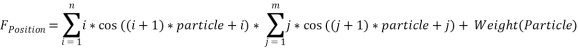10: Minimum (F_Position)

11: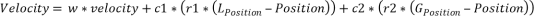12: Position = Position + Velocity

13: For k = 1 to iteration

If Present_fitness < Last_fitness

Update fitness value

End If

Update velocity and position.

End For

14: Find maximum (fitness_value), mf = max (fitness)

15: Place PMU on that bus.

16: Update binary table by eliminating the bus from binary table.

17: Loop to Step 6 until binary table gets empty.

18: If the PMU placed at only one bus,

Check the nearest bus and made connection between them and update cluster.

19: End If

The proposed algorithm is applied on the three cases for optimal PMU placement.

## B. Case 1: Without PMU Loss

In this case, the zero injection and the flow measurement are ignored. To formulate the constraint set, the binary connectivity matric is formed whose entries are defined in the following equation: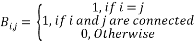(1)

The matrix can be directly calculated from the bus admittance matrix by converting the entries in the binary form.

Consider the six bus system

The binary table B is defined as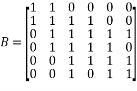(2)

The constraints for this case is,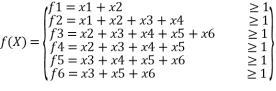(3)

From the binary table, identify the maximum connectivity among the buses. The table shows the maximum connectivity is occurred in bus 3. Hence, bus 2, 3, 4, 5, and 6 are eliminated from the binary table.

Then, the binary table can be updated as,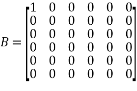(4)

After performing the PSO based clustering algorithm, the PMU is placed on bus 1 and bus 3, which is shown in fig.3.

## C. Case 2: With loss of PMU

It is considered as each bus is observable by single PMU and these PMUs are placed by the proposed clustering algorithm. Hence, the placement of PMUs are highly reliable but, if any disturbance occurred in power system or due to maintenance purpose any of the PMUs places is out from the system. If any of the PMU is disconnected, then some of the buses are connected to that PMUs are not remain observable. In order to overcome such unexpected PMU failures, a strategy is considered for single PMU loss. It can be achieved if all the buses are observed by at least two PMUs. These are operated as two sets,

1. Primary set
2. Backup set

If suppose the PMU from primary set is not working properly, then the backup set will take the responsibility to observe the buses. To obtain the couple of PMUs, the constraint and objective function will remain same by only modifying the change in matrix f. In this case, the elements of f is equal to 2 instead of 1. It is defined as follows: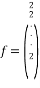(5)

This case place the PMU for monitoring the single bus by two PMUs. Other than the objective function, the steps are same. The new constraint function can be constructed as follows: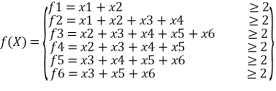(6)

## D. Case 3: Zero Injection

Zero injection buses are the buses from that no current is passed into the system. Zero injection correspond to the transferring nodes in the system. If zero injection buses are also designed in the PMU placement problem, the entire number of PMUs are further minimized. Consider the following example for zero injection on six bus system where bus 2 is considered as the zero injection bus.

Now, the constraint for zero injection bus can be written as follows,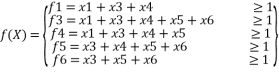(7)

From the above equation, it is identified that the bus 3 has maximum connectivity. Hence, PMU is placed on the bus for entire system observability.

## IV. Performance Analysis

To evaluate the performance of the proposed method, the optimal placement of PMU problem is solved for IEEE standard bus system and KPTCL 220 and 400 kV power systems. The KPTCL power buses are shown in fig.6. The results of the proposed method for IEEE bus system is illustrated in table 2. Here, IEEE-6 bus, IEEE-7 bus, IEEE-9 bus, IEEE-14 bus, and IEEE-30 bus system are considered for evaluation. Table 2 provides results for the three cases of IEEE bus systems.

We collect the data from the KPTCL 220 and 400 kV power system. Here, the PMU placement is obtained only for the case 1 (without PMU loss). Hence, we proposed an algorithm to obtain the PMU placement, which suits for all the three cases (with loss, without loss, zero injection bus). Table 3 provides the total number of PMU placement collected from the KPTCL. Whereas table 4 provides the proposed result for the given power system. The proposed method results for 28 bus, 127 bus and 155 bus system in all the three cases.

## V. Conclusion and Future Work

In this paper, a PSO based cluster formation algorithm is proposed to solve the optimal PMU placement problem.

## Related Services

View all

### DMCA / Removal Request

If you are the original writer of this essay and no longer wish to have your work published on the UKDiss.com website then please:

Related Services